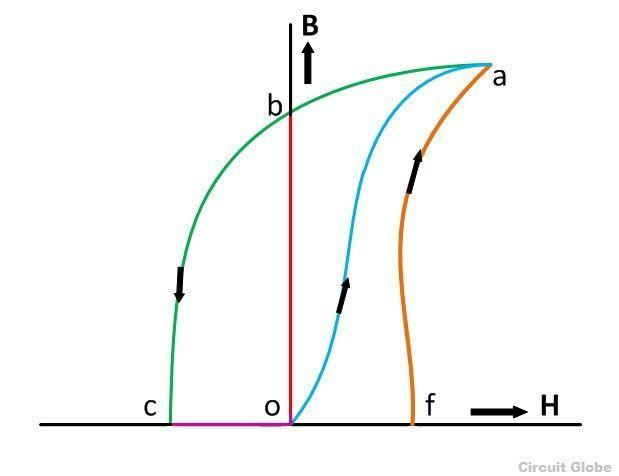Home Technology Residual magnetization

# Residual magnetization

## Introduction

Remanence (Remanence) symbol is Br, which means that after the magnet is magnetized to saturation, the external magnetic field is removed. It can still maintain a certain degree of magnetization in the original direction of the external magnetic field. The limit value of remanence is the saturation magnetization. The remanence of permanent magnetic materials is mainly affected by the orientation of each crystal grain and the magnetic domain structure in the material.

Remanence can be used to measure the degree of magnetization. When the magnet is magnetized, there is remanence. Magnetic storage equipment uses remanence to record data. When studying the earth's magnetic field in paleomagnetism, remanence can also provide a lot of information.

In engineering applications other than magnets, residual magnetization is also called residual magnetization (residual magnetization). Such as transformers, motors, generators and electromagnetic steel generally do not want to have too much remanence, because remanence will bring undesirable results. For example, if the electromagnet has remanence, it will still have magnetism when the coil is not conducting. Remanence can be removed by demagnetization.

The unit of remanence in the international system of units is Tesla (T), and the unit of the CGS Gauss system is Gauss (G), 1T = 10000G.## Classification of remanence

### Saturation remanence

The definition of remanence is to remove the magnetic field after applying a magnetic field (it needs to be large enough to produce magnetic saturation) In addition, the remaining magnetization. The hysteresis curve can be measured with a device such as a vibrating sample magnetometer. The point where the magnetic field is 0 is the remanence. Physically the residual magnetization will be converted into the average magnetization (magnetic moment divided by the total volume), which is represented by Mr. If it needs to be distinguished from other remanence, this remanence will be called saturation remanence (saturation remanence) or saturation isothermal remanence (saturation isothermal remanence, SIRM), The symbol is Mrs.

In engineering applications, the B-H analyzer is used to measure the remanence. The B-H analyzer measures the response under an AC magnetic field. Residual magnetism is the most important characteristic of permanent magnets, indicating the maximum magnetization that permanent magnets can produce. For example, the remanence of a neodymium magnet is about 1.3 Tesla.

### Isothermal remanence

The data of a remanence cannot fully represent the characteristics of the magnet. For example, there are many small magnetic particles in magnetic tape (see magnetic storage), and these particles are not exactly the same. Magnets in rocks also have different magnetic properties (see Rock Magnetism). To further understand its characteristics, you can increase or decrease the remanence. One way is to demagnetize the magnet with an AC magnetic field, and then apply a magnetic field H before removing it. This kind of remanence is represented by Mr(H), which is related to the size of the magnetic field, and is called initial remanence or isothermal remanent magnetization (IRM).

Another way to measure isothermal remanence is to first saturate the magnet in one direction, and then apply a magnetic field in the opposite direction before removing it. This is called demagnetization remanence (DC demagnetization remanence) and is represented by Md(H), where H is the size of the magnetic field. Another way is to demagnetize the saturated magnet in an AC magnetic field, called AC demagnetization remanence (AC demagnetization remanence, alternating field demagnetization remanenc), which is represented by the symbol Maf(H).

If the particles are single magnetic domain particles that do not affect each other and have uniaxial anisotropy, there will be a simple linear relationship between the above remanence.

## Non-hysteresis remanence

Anhysteretic remanent magnetization (ARM) is another common type of remanence in the laboratory. A small DC magnetic field is added to the magnetic field. The size of the AC magnetic field gradually decreases, and finally drops to zero, to obtain anhysteretic magnetization (anhysteretic magnetization), and then remove the magnetic field to obtain remanence. The curve of non-hysteresis remanence is mostly close to the average value on both sides of the hysteresis curve, and it is assumed in some models that it can represent the lowest energy state of a specific magnetic field. Non-hysteresis remanence is also similar to the writing process under some magnetic recording technologies, and the natural remanence in rocks.

## Related items

• Coercivity

• Hysteresis and hysteresis

< /li>